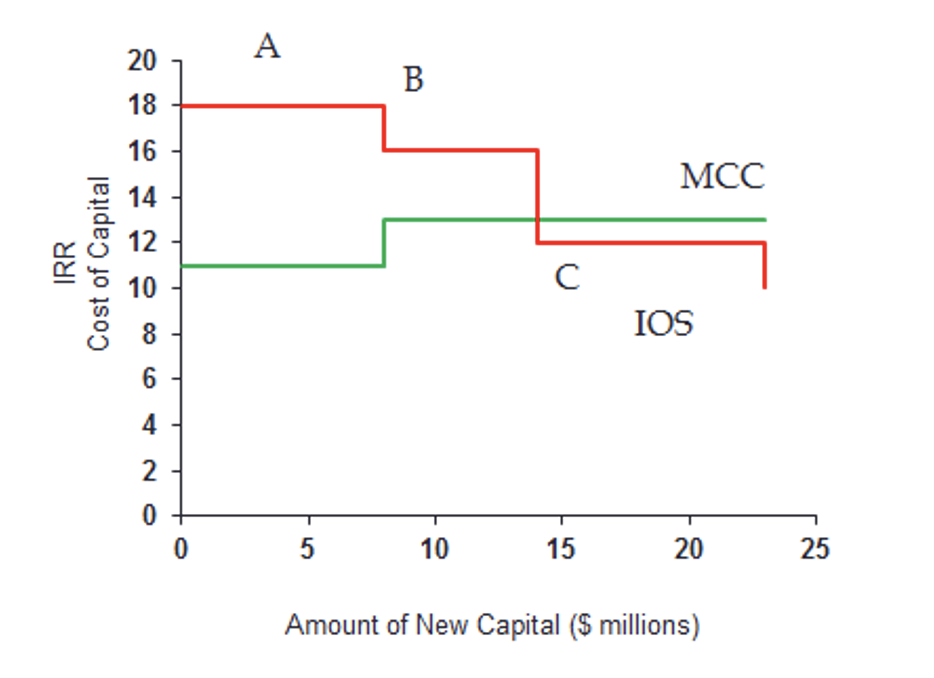# Applications of Cost of Capital

The marginal cost of capital plays an important role in capital budgeting and investment decisions.

As a firm raises more and more capital, it's marginal cost of capital (MCC) increases. However, when a firm makes more investments, the returns from additional investments decrease. This is represented by the investment opportunity schedule (IOS).

The MCC schedule represents the cost of capital faced by the firm (ranking from the cheapest to the most expensive) while the IOS represents the projects that are available to the firm (ranking from the most

In order to construct the IOS, the firm needs to first estimate the IRR of each of the project it is considering. Once that is accomplished, the financial manager can plot the IOS, which is a chart of the IRRs of the firm’s projects arranged from the highest IRR to the lowest IRR.

The MCC curve is upward sloping while the IOS curve is downward sloping.The MCC breaks upward when the cost of a capital component increases. The first break usually occurs when retained earnings are exhausted and outside capital is raised at higher cost.

With respect to the investment decisions, the company can achieve optimal capital budget, which is the amount of capital at which the firms marginal cost of capital is equal to the marginal returns from investing.

While valuing individual projects, the company's WACC is used in the calculation of the project's NPV. If the NPV is positive, the company invests in the project otherwise not. When WACC is used in the calculation of a project's NPV, there are a few implicit assumptions such as the project has the same risk as the average risk of the company, and the firm's capital structure remains the same throughout the life of the project. This is because if the capital structure changes, the WACC will fluctuate.

The logic behind using WACC as the discount rate for all projects is that all the sources of funds must be repaid regardless of which project is generating the cash flow. This is regardless of the incremental funding source for the project being considered.

An analyst can also use WACC for valuing securities, using many of the DCF approaches. If the cash flows are the free cash flows for the firm, the the analyst uses WACC to calculate the present value of cash flows. If, however, the cash flows are specifically for the company's owners, then the analyst uses cost of capital.